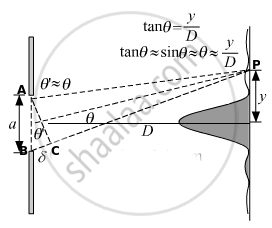# Derive the relation a sin θ = λ for the first minimum of the diffraction pattern produced due to a single slit of width 'a' using light of wavelength λ. - Physics

Numerical

Derive the relation a sin θ = λ for the first minimum of the diffraction pattern produced due to a single slit of width 'a' using light of wavelength λ.

#### SolutionAs we can see from the figure, rays AP and BP reach to the screen from the two ends of a single slit. The ray BP travels an extra distance of BC as compared to ray AP.

Hence the path difference produced, Δx = BC ..........(1)

The ray reaching to screen at point P from point O makes the angle θ with the horizontal.

∵ ∠POQ = θ

∴∠BAC = θ

In ΔABC,

BC = a sin θ

Using equation (1), Δx = BC

⇒ Δx = a sin θ  .............(2)

For diffraction pattern produced due to a single slit of width, 'a' using the light of wavelength 'λ''λ', path difference for destructive interference is given by, Δx = mλ (where m = 1, -1, 2, -2, 3, -3, 4..............)

Using equation (2), we can write,  a sin θ = mλ

For the first minimum,

m = 1,

∴ a sin θ  = λ

Concept: Diffraction - Seeing the Single Slit Diffraction Pattern
Is there an error in this question or solution?